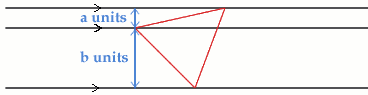You may also likeDiophantine N-tuples

Can you explain why a sequence of operations always gives you perfect squares?DOTS Division

Take any pair of two digit numbers x=ab and y=cd where, without loss of generality, ab > cd . Form two 4 digit numbers r=abcd and s=cdab and calculate: {r^2 - s^2} /{x^2 - y^2}.Sixational

The nth term of a sequence is given by the formula n^3 + 11n . Find the first four terms of the sequence given by this formula and the first term of the sequence which is bigger than one million. Prove that all terms of the sequence are divisible by 6.

Pareq Calc

Age 14 to 16Challenge LevelTriangle ABC is an equilateral triangle and the three lines marked with arrows in the diagram are parallel. If the perpendicular distances between the parallel lines are 1 unit and 2 units then calculate the length of the sides of the triangle. Suppose the distances between the parallel lines are a units and b units then what is the length of the sides of the triangle?